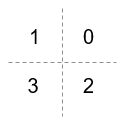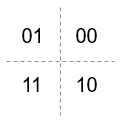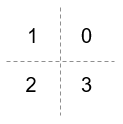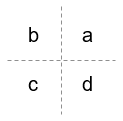# comm.OQPSKModulator

Modulation using OQPSK method

## Description

The comm.OQPSKModulator object modulates the input signal using the offset quadrature phase shift keying (OQPSK) method and applies pulse shape filtering to the output waveform. For more information, see Pulse Shaping Filter. The output is a baseband representation of the modulated signal.

For information about delays incurred by modulator-demodulator pair processing, see Modulation Delays.

To modulate a signal using offset quadrature phase shift keying:

1. Create the `comm.OQPSKModulator` object and set its properties.

2. Call the object with arguments, as if it were a function.

## Creation

### Syntax

``oqpskmod = comm.OQPSKModulator``
``oqpskmod = comm.OQPSKModulator(demod)``
``oqpskmod = comm.OQPSKModulator(Name,Value)``
``oqpskmod = comm.OQPSKModulator(phase,Name,Value)``

### Description

````oqpskmod = comm.OQPSKModulator` creates a modulator System object™. This object applies offset quadrature phase shift keying (OQPSK) modulation and pulse shape filtering to the input signal.```

example

````oqpskmod = comm.OQPSKModulator(demod)` creates a modulator System object with symmetric configuration to the OQPSK demodulator object, `demod`.```

example

````oqpskmod = comm.OQPSKModulator(Name,Value)` sets properties using one or more name-value pairs. Enclose each property name in single quotes.Example: `comm.OQPSKModulator('BitInput',true)````
````oqpskmod = comm.OQPSKModulator(phase,Name,Value)` sets the PhaseOffset property of the created object to `phase` and sets any other specified `Name`, `Value` pairs.Example: `comm.OQPSKModulator(0.5*pi,'SymbolMapping','Binary')````

## Properties

expand all

Unless otherwise indicated, properties are nontunable, which means you cannot change their values after calling the object. Objects lock when you call them, and the `release` function unlocks them.

If a property is tunable, you can change its value at any time.

Phase of zeroth point of the signal constellation in radians, specified as a scalar.

Example: `'PhaseOffset',0` aligns the OQPSK signal constellation points on the axes, {(1,0), (0,j), (-1,0), (0,-j)}.

Data Types: `double`

Option to provide input in bits, specified as `false` or `true`.

• When this property is set to `false`, the input values must be integer representations of two-bit input segments and range from 0 to 3.

• When this property is set to `true`, the input must be a binary vector of even length. Element pairs are binary representations of integers.

Data Types: `logical`

Signal constellation bit mapping, specified as `'Gray'`, `'Binary'`, or a custom 4-element numeric vector of integers with values from 0 to 3.

SettingConstellation Mapping for IntegersConstellation Mapping for BitsComment

`Gray`The signal constellation mapping is Gray-encoded.

`Binary`The signal constellation mapping for the input integer `m` (0 ≤ `m` ≤ 3) is the complex value ```e(j*(PhaseOffset+π/4) + j*2*π*m/4)```.

Custom 4-element numeric vector of integers with values from 0 to 3Elements [a b c d] must be composed of the set of values [0, 1, 2, 3] in any order.

Data Types: `char` | `double`

Filtering pulse shape, specified as `'Half sine'`, ```'Normal raised cosine'```, `'Root raised cosine'`, or `'Custom'`.

Data Types: `char`

Raised cosine filter rolloff factor, specified as a scalar from `0` to `1`.

#### Dependencies

This property applies when PulseShape is `'Normal raised cosine'` or ```'Root raised cosine'```.

Data Types: `double`

Filter length in symbols, specified as a scalar. An ideal raised cosine filter has an infinite impulse response. However, to realize a practical implementation of this filter, the object truncates the impulse response to `FilterSpanInSymbols` symbols.

#### Dependencies

This property applies when PulseShape is `'Normal raised cosine'` or ```'Root raised cosine'```.

Data Types: `double`

Filter numerator, specified as a row vector.

#### Dependencies

This property applies when PulseShape is `'Custom'`.

Data Types: `double`
Complex Number Support: Yes

Number of samples per symbol, specified as a positive even integer.

Data Types: `double`

Data type assigned to output, specified as `'double'` or `'single'`.

Data Types: `char`

## Usage

### Syntax

``waveform = oqpskmod(insignal)``

### Description

example

````waveform = oqpskmod(insignal)` returns baseband-modulated output. The output waveform is pulse shaped according to the configuration properties PulseShape and SamplesPerSymbol.```

### Input Arguments

expand all

Input signal, specified as an NS-element column vector of integers or bits, where NS is the number of samples.

The setting of the BitInput property determines the interpretation of the input vector.

Data Types: `double`

### Output Arguments

expand all

Output waveform, returned as a vector. The output waveform is pulse-shaped according to the configuration properties PulseShape and SamplesPerSymbol.

## Object Functions

To use an object function, specify the System object as the first input argument. For example, to release system resources of a System object named `obj`, use this syntax:

`release(obj)`

expand all

 `step` Run System object algorithm `release` Release resources and allow changes to System object property values and input characteristics `reset` Reset internal states of System object

## Examples

collapse all

Create an OQPSK modulator and demodulator pair. Create an AWGN channel object having two bits per symbol.

```oqpskmod = comm.OQPSKModulator('BitInput',true); oqpskdemod = comm.OQPSKDemodulator('BitOutput',true); channel = comm.AWGNChannel('EbNo',4,'BitsPerSymbol',2);```

Create an error rate calculator. To account for the delay between the modulator and demodulator, set the `ReceiveDelay` property to `2`.

`errorRate = comm.ErrorRate('ReceiveDelay',2);`

Process 300 frames of data looping through these steps.

• Generate vectors with 100 elements of random binary data.

• OQPSK-modulate the data. The data frames are processed as 50 sample frames of 2-bit binary data.

• Pass the modulated data through the AWGN channel.

• OQPSK-demodulate the data.

• Collect error statistics on the frames of data.

```for counter = 1:300 txData = randi([0 1],100,1); modSig = oqpskmod(txData); rxSig = channel(modSig); rxData = oqpskdemod(rxSig); errorStats = errorRate(txData,rxData); end```

Display the error statistics.

`ber = errorStats(1)`
```ber = 3.3336e-05 ```
`numErrors = errorStats(2)`
```numErrors = 1 ```
`numBits = errorStats(3)`
```numBits = 29998 ```

Use an OQPSK demodulator object to initialize an OQPSK modulator object while creating it.

Create an OQPSK demodulator, assigning it a phase offset of $\frac{1}{2}\pi$.

```phase = 0.5*pi; oqpskdemod = comm.OQPSKDemodulator(phase)```
```oqpskdemod = comm.OQPSKDemodulator with properties: Modulation PhaseOffset: 1.5708 SymbolMapping: 'Gray' BitOutput: false Filtering PulseShape: 'Half sine' SamplesPerSymbol: 4 OutputDataType: 'double' ```

Use the demodulator object to initialize an OQPSK modulator while creating it.

`oqpskmod = comm.OQPSKModulator(oqpskdemod)`
```oqpskmod = comm.OQPSKModulator with properties: Modulation PhaseOffset: 1.5708 SymbolMapping: 'Gray' BitInput: false Filtering PulseShape: 'Half sine' SamplesPerSymbol: 4 OutputDataType: 'double' ```

Perform OQPSK modulation and demodulation and apply root raised cosine filtering to a waveform.

System initialization

Define simulation parameters and create objects for OQPSK modulation and demodulation.

```sps = 12; % samples per symbol bits = randi([0, 1], 800, 1); % transmission data modulator = comm.OQPSKModulator('BitInput',true,'SamplesPerSymbol',sps,'PulseShape','Root raised cosine'); demodulator = comm.OQPSKDemodulator(modulator);```

Waveform transmission and reception

Use the `modulator` object to apply OQPSK modulation and transmit filtering to the input data.

```oqpskWaveform = modulator(bits); ```

Pass the waveform through a channel.

```snr = 0; rxWaveform = awgn(oqpskWaveform, snr);```

Use the `demodulator` object to apply receive filtering and OQPSK demodulation to the waveform.

```demodData = demodulator(rxWaveform); ```

Compute the bit error rate to confirm the quality of the data recovery.

```delay = (1+modulator.BitInput)*modulator.FilterSpanInSymbols; [~, ber] = biterr(bits(1:end-delay), demodData(delay+1:end))```
```ber = 0 ```

Use the `qamdemod` function to simulate soft decision output for OQPSK-modulated signals.

Generate an OQPSK modulated signal.

```sps = 4; msg = randi([0 1],1000,1); oqpskMod = comm.OQPSKModulator('SamplesPerSymbol',sps,'BitInput',true); oqpskSig = oqpskMod(msg);```

Add noise to the generated signal.

`impairedSig = awgn(oqpskSig,15);`

Perform Soft-Decision Demodulation

Create QPSK equivalent signal to align in-phase and quadrature.

`impairedQPSK = complex(real(impairedSig(1+sps/2:end-sps/2)), imag(impairedSig(sps+1:end)));`

Apply matched filtering to the received OQPSK signal.

```halfSinePulse = sin(0:pi/sps:(sps)*pi/sps); matchedFilter = dsp.FIRDecimator(sps,halfSinePulse,'DecimationOffset',sps/2); filteredQPSK = matchedFilter(impairedQPSK);```

To perform soft demodulation of the filtered OQPSK signal use the `qamdemod` function. Align symbol mapping of `qamdemod` with the symbol mapping used by the `comm.OQPSKModulator`, then demodulate the signal.

```oqpskModSymbolMapping = [1 3 0 2]; demodulated = qamdemod(filteredQPSK,4,oqpskModSymbolMapping,'OutputType','llr');```

expand all